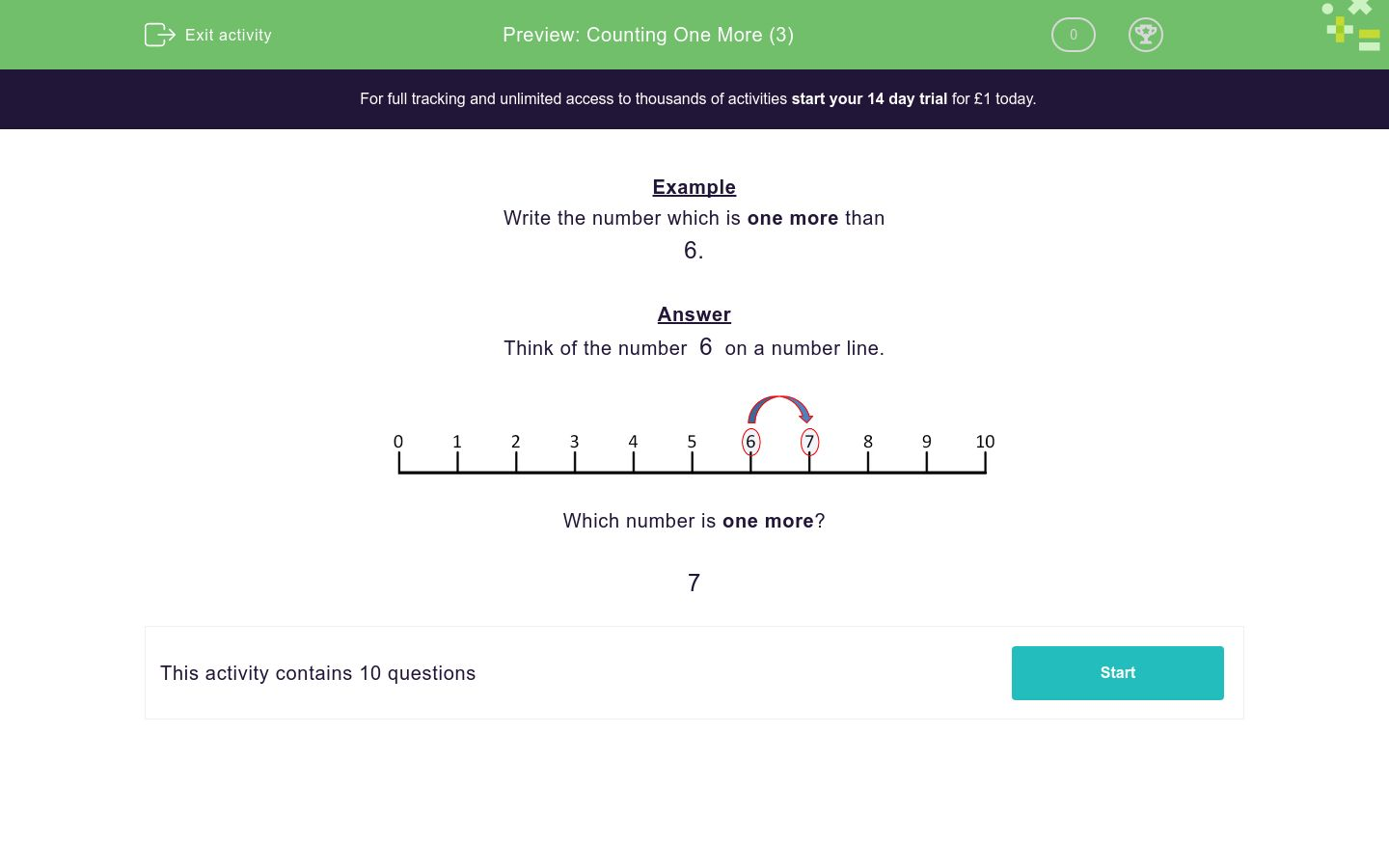# Counting One More (3)

In this worksheet, children must write the number that is one more than the number shown.Key stage:  KS 1

Curriculum topic:   Number: Number and Place Value

Curriculum subtopic:   Identify One More/One Less

Difficulty level:### QUESTION 1 of 10

Example

Write the number which is one more than

6.

Think of the number  6  on a number line.Which number is one more?

7

Write the number which is one more than

3

Write the number which is one more than

1

Write the number which is one more than

0

Write the number which is one more than

7

Write the number which is one more than

2

Write the number which is one more than

4

Write the number which is one more than

9

Write the number which is one more than

8

Write the number which is one more than

6

Write the number which is one more than

5

• Question 1

Write the number which is one more than

3

4
EDDIE SAYS
0... 1... 2... 3 and then... 4
• Question 2

Write the number which is one more than

1

2
EDDIE SAYS
0... 1 and then... 2
• Question 3

Write the number which is one more than

0

1
EDDIE SAYS
0 and then... 1
• Question 4

Write the number which is one more than

7

8
EDDIE SAYS
0... 1... 2... 3... 4... 5... 6... 7 and then... 8
• Question 5

Write the number which is one more than

2

3
EDDIE SAYS
0... 1... 2 and then... 3
• Question 6

Write the number which is one more than

4

5
EDDIE SAYS
0... 1... 2... 3... 4 and then... 5
• Question 7

Write the number which is one more than

9

10
EDDIE SAYS
0... 1... 2... 3... 4... 5... 6... 7... 8... 9 and then... 10
• Question 8

Write the number which is one more than

8

9
EDDIE SAYS
0... 1... 2... 3... 4... 5... 6... 7... 8 and then... 9
• Question 9

Write the number which is one more than

6

7
EDDIE SAYS
0... 1... 2... 3... 4... 5... 6 and then... 7
• Question 10

Write the number which is one more than

5

6
EDDIE SAYS
0... 1... 2... 3... 4... 5 and then... 6
---- OR ----

Sign up for a £1 trial so you can track and measure your child's progress on this activity.

### What is EdPlace?

We're your National Curriculum aligned online education content provider helping each child succeed in English, maths and science from year 1 to GCSE. With an EdPlace account you’ll be able to track and measure progress, helping each child achieve their best. We build confidence and attainment by personalising each child’s learning at a level that suits them.

Get started